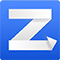# excel函数中3个常用的公式优化方法【例1】利用vlookup查找
=vlookup(A1,B:D,2,0)

=vlookup(A1,B:D,2,)

【例2】把A1单元格中字符A替换为空
=SUBSTITUTE(A1,"A","")

=SUBSTITUTE(A1,"A",)

【例3】乘10000
=A1*10000

=A1/1%%

【例4】除100
=A1/100

=A1%

【例5】如果A1值大于200,结果是200，否则还是A1的值
=IF(A1>200,200,A1)

=Min(A1,200)

【例6】如果从表1查找的值大于100，则显示“超额完成”，等于100返回“持平”，小于100则返回"未完成"
=IF(Vlookup(a1,表1!A:D,3,0)>100,"完成",Vlookup(a1,表1!A:D,3,0)=100,"持平","未完成")

=TEXT(VLOOKUP(A1,表1!A:D,3,0)-100,"完成;未完成;持平")